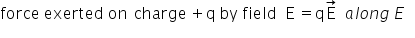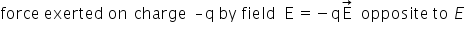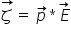# Torque on a dipole

Torque on a Dipole in a Uniform Field:

consider an electric dipole consisting of charges +q and –q and of length 2a placed in a uniform electric field E making an angle θ with it. It has a dipole moment of magnitude, p=q*2aFtotal = 0

Hence the met translating force o a dipole in a uniform electric field is zero. But the two equal and opposite forces act at different points of the dipole they form a couple which exerts a torque.

Torque = either charge*perpendicular distance between the two forces

τ=qE * 2a sin θ

As the direction of torque is perpendicular  to both P and E. so we can writeClearly, the torque on the dipole will be maximum when the dipole is held perpendicular to E. Thus

τ max = PE sin θ = PE

and minimum when angle is 00, when the dipole get aligned with E ,the torque becomes zero.

τ min = PE sin 0 = 0

Related Keywords